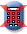Massachusetts Comprehensive Assessment SystemReporting Category: Algebra and Functions
Standard: 10.P.1 - Describe, complete, extend, analyze, generalize, and create a wide variety of patterns, including iterative, recursive (e.g., Fibonacci Numbers), linear, quadratic, and exponential functional relationships. (AI.P.1)
Standard: CCSS.Math.Content.HSF-LE.A.2 - Construct linear and exponential functions, including arithmetic and geometric sequences, given a graph, a description of a relationship, or two input-output pairs (include reading these from a table).*

Lionel wrote an arithmetic sequence. The first five terms of the sequence are shown below.

3, 10, 17, 24, 31, . . .

1. What is the next term of Lionel's sequence? Show or explain how you got your answer.
2. Write an expression that can be used to find the nth term of Lionel's sequence.

Bella also wrote an arithmetic sequence. Each term of Bella's sequence is equal to three times the corresponding term of Lionel's sequence.

1. Write an expression that can be used to find the mth term of Bella's sequence.
2. What is the 20th term of Bella's sequence? Show or explain how you got your answer.

## Scoring Guide and Sample Student Work

Select a score point in the table below to view the sample student response.

ScoreDescription
4 The student response demonstrates an exemplary understanding of the Algebra and Functions concepts involved in writing arithmetic sequences with an explicit formula and using them to model situations. The student writes an expression that represents an arithmetic sequence and uses that expression to extend the sequence.
4
3 The student response demonstrates a good understanding of the Algebra and Functions concepts involved in writing arithmetic sequences with an explicit formula and using them to model situations. Although there is significant evidence that the student was able to recognize and apply the concepts involved, some aspect of the response is flawed. As a result the response merits 3 points.
2 The student response demonstrates a fair understanding of the Algebra and Functions concepts involved in writing arithmetic sequences with an explicit formula and using them to model situations. While some aspects of the task are completed correctly, others are not. The mixed evidence provided by the student merits 2 points.
1 The student response demonstrates a minimal understanding of the Algebra and Functions concepts involved in writing arithmetic sequences with an explicit formula and using them to model situations.
0 The student response contains insufficient evidence of an understanding of the Algebra and Functions concepts involved in writing arithmetic sequences with an explicit formula and using them to model situations to merit any points.
Note: There are 2 sample student responses for Score Point 4.

Question 17: Number and Quantity
Question 20: Algebra and Functions
Question 21: Algebra and Functions
Question 36: Geometry
Question 41: Statistics and Probability
Question 42: Geometry

Last Updated: April 5, 2022